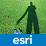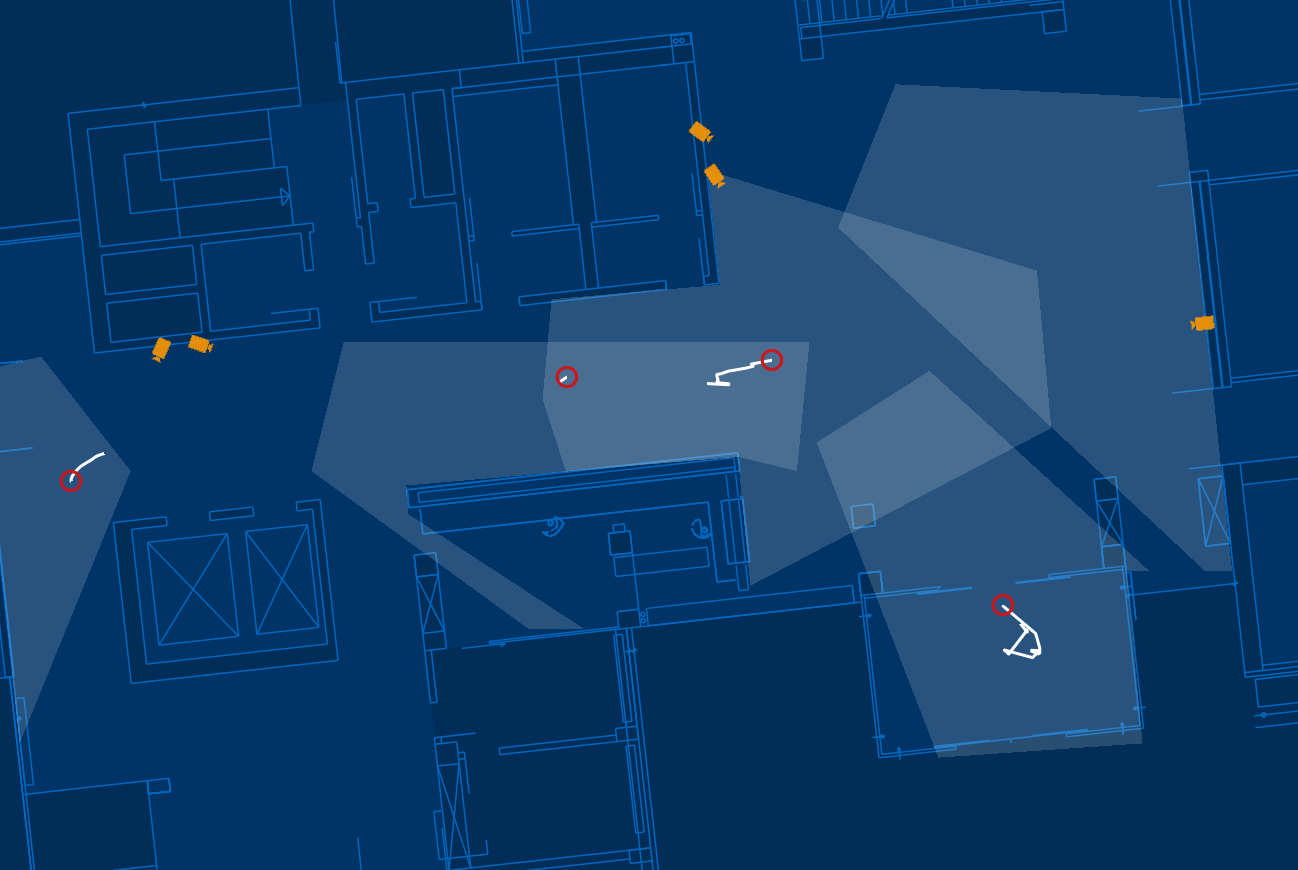# Way to get onScreen coordinates of all visible Graphics

2617
5
08-19-2014 01:51 AMNew Contributor

Is there an easy way to get the onScreen-coordinates of all visible Graphics objects?

1 Solution

Accepted SolutionsOccasional Contributor

Another thing you could try would be to use a 'hit test' on the graphics layers to find all graphics within a certain pixel tolerance of a screen point.

One overload of graphicsLayer.getGraphicIDs takes an x, y, as well as a pixel tolerance and max number of results. The tolerance is a square around the x, y point (such that a side of the square is 2*tolerance). You could set the max results to something high (depending on how many graphics you're expecting in your extent at one time) and the tolerance to be for example 1/2 the max(extentHeight, extentWidth). You could at least use this to reduce the number of graphics you're checking against the 'contains' in your code above.

~elise

5 RepliesOccasional Contributor

Do you mean visible as in whether it's in the current map extent?

And what have you tried so far?

~eliseNew Contributor

Yes I meant the current map extent, like in this screenshot. The Icons are in different GraphicsLayer.The method I tried is to iterate over all graphics layer, iterate over all graphics, check if the geometry is in the current extent and then call the JMap.toScreenPoint() method. Here is a snippet of the code:

List<Point> coords = new ArrayList<Point>();

LayerList list = map.getLayers();

for (int i = list.size() - 1; i >= 0; i--) {

if (list.get(i) instanceof GraphicsLayer) {

GraphicsLayer layer = (GraphicsLayer) list.get(i);

if (layer.getGraphicIDs() != null && layer.getGraphicIDs().length > 0) {

int[] ids = layer.getGraphicIDs();

for (int j = 0; j < ids.length; j++) {

Graphic graphic = layer.getGraphic(ids);

com.esri.core.geometry.Geometry geometry = graphic.getGeometry();

if (com.esri.core.geometry.Geometry.isPoint(geometry.getType().a())) {

Point p = (Point) geometry;

Envelope extent = map.getExtent();

if (extent.contains(p)) {

Point screenPoint = map.toScreenPoint(p);

}

}

}

}

}

This works, but i hope there is an easier and perhaps faster way. Particularly for other geometry objects like polylines and polygons.

I need to call this method after every change of the map extent or change in the data.Occasional Contributor

Another thing you could try would be to use a 'hit test' on the graphics layers to find all graphics within a certain pixel tolerance of a screen point.

One overload of graphicsLayer.getGraphicIDs takes an x, y, as well as a pixel tolerance and max number of results. The tolerance is a square around the x, y point (such that a side of the square is 2*tolerance). You could set the max results to something high (depending on how many graphics you're expecting in your extent at one time) and the tolerance to be for example 1/2 the max(extentHeight, extentWidth). You could at least use this to reduce the number of graphics you're checking against the 'contains' in your code above.

~eliseNew Contributor

My final solution is this:

List<Point> coords = new ArrayList<Point>();

LayerList list = map.getLayers();

for (int i = list.size() - 1; i >= 0; i--) {

if (list.get(i) instanceof GraphicsLayer) {

GraphicsLayer layer = (GraphicsLayer) list.get(i);

int[] graphicIDs = layer.getGraphicIDs(map.getWidth() / 2, map.getHeight() / 2, Math.max(map.getWidth(), map.getHeight())/2);

if (graphicIDs != null && graphicIDs.length > 0) {

int[] ids = graphicIDs;

for (int j = 0; j < ids.length; j++) {

Graphic graphic = layer.getGraphic(ids);

com.esri.core.geometry.Geometry geometry = graphic.getGeometry();

if (com.esri.core.geometry.Geometry.isPoint(geometry.getType().a())) {

Point p = (Point) geometry;

Envelope extent = map.getExtent();

if (extent.contains(p)) {

Point screenPoint = map.toScreenPoint(p);

}

}

}

}

}

}

This works, but with with over 10000 objects on screen it takes a lot of time. It would be nice if the javadoc for getGraphicIDs(float x, float y, int tolerance) mentioned that the search tolerance is used for the sides of a square and not as radius for a circle.Occasional Contributor

Yes I completely agree about the doc and actually I'd gone ahead and added that info to these 'hit test' methods, so it will be there at the next release.

In your code you might want to just do a type check for Point (i.e., if (geometry instanceof Point)) before you cast it to Point. Calling isPoint on the geometry will return true if the geometry "is a point type (dimension 0)", so actually it's not a guarantee it's of the class Point - for example it could be of the class MultiPoint in which case you'd get a ClassCastException.

~elise